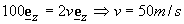EXAMPLE 3: THE ANGULAR IMPULSE-MOMENTUM RELATIONThe motor M applies a constant moment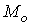to rotate the particle. If the particle of mass m=20 kg starts from rest and the motor applies a moment=10 N-m, calculate the speed of the particle after 10 seconds. Assume the particle is fixed to the bar at a radius of 0.1 m.

Solution: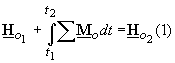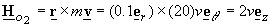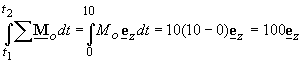substitution into (1) gives